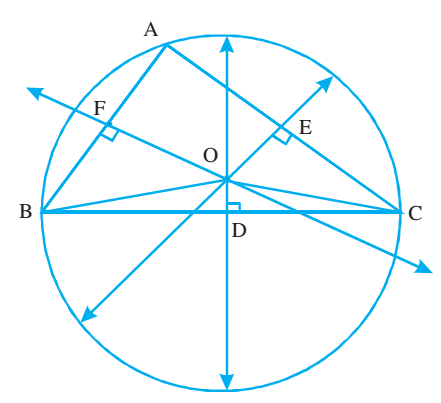# Perpendicular Bisectors of Sides of a Triangle

A line which bisects a side of a triangle at right angle is called the perpendicular bisector of the side. Since a triangle has three sides, you can draw three perpendicular bisectors in a triangle.

The three perpendicular bisectors of the sides of a triangle pass through the same point, that is, they are concurrent. The point of concurrency O is called the circumcentre of the triangle.If we take O as the centre and AO as the radius, you can draw a circle passing through the three vertices, A, B and C of the triangle, called Circumcircle of the triangle. O being the centre of this circle is called the circumcentre and AO the radius of the circumcircle is called circumradius of the triangle.

The circumcentre will be

1. in the interior of the triangle for an acute triangle
2. on the hypotenuse for a right triangle
3. in the exterior of the triangle for an obtuse triangle